﻿ Mechanics Map - Equilibrium Analysis for Concurrent Force Systems

# Equilibrium Analysis for Concurrent Force Systems

If a body is in static equilibrium, then by definition that body is not accelerating. If we know that the body is not accelerating then we know that the sum of the forces acting on that body must be equal to zero. This is the basis for equilibrium analysis for a particle.

In order to solve for any unknowns in our sum of forces equation, we actually need to turn the one vector equation into a set of scalar equations. For two dimensional problems, we will split our one vector equation down into two scalar equations. We do this by summing up all the x components of the force vectors and setting them equal to zero in our first equation, and summing up all the y components of the force vectors and setting them equal to zero in our second equation.

 $\sum \vec{F}=0$ $\sum F_x=0$ $\sum F_y=0$

We do something similar in three dimensional problems except we will break all our force vectors down into x, y, and z components, setting the sum of x components equal to zero for our first equation, the sum of all the y components equal to zero for our second equation, and the sum of all our z components equal to zero for our third equation.

 $\sum \vec{F}=0$ $\sum F_x=0$ $\sum F_y=0$ $\sum F_z=0$

Once we have written out the equilibrium equations, we can solve the equations for any unknown forces.

## Finding the Equilibrium Equations:

The first step in finding the equilibrium equations is to draw a free body diagram of the body being analyzed. This diagram should show all the known and unknown force vectors acting on the body. In the free body diagram, provide values for any of the know magnitudes or directions for the force vectors and provide variable names for any unknowns (either magnitudes or directions).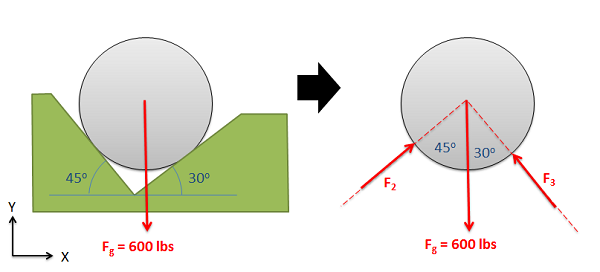The first step in equilibrium analysis is drawing a free body diagram. This is done by removing everything but the body and drawing in all forces acting on the body. It is also useful to label all forces, key dimensions, and angles.

Next you will need to choose the x, y, and z axes. These axes do need to be perpendicular to one another, but they do not necessarily have to be horizontal or vertical. If you choose coordinate axes that line up with some of your force vectors you will simplify later analysis.

Once you have chosen axes, you need to break down all of the force vectors into components along the x, y and z directions (see the vectors page in Appendix 1 if you need more guidance on this). Your first equation will be the sum of the magnitudes of the components in the x direction being equal to zero, the second equation will be the sum of the magnitudes of the components in the y direction being equal to zero, and the third (if you have a 3D problem) will be the sum of the magnitudes in the z direction being equal to zero. Collectively these are known as the equilibrium equations.

Once you have your equilibrium equations, you can solve them for unknowns using algebra. The number of unknowns that you will be able to solve for will be the number of equilibrium equations that you have. In instances where you have more unknowns than equations, the problem is known as a statically indeterminate problem and you will need additional information to solve for the given unknowns.

## Worked Problems:

### Question 1:

A 6 kg traffic light is supported by two cables as shown below. Find the tension in each of the cables supporting the traffic light.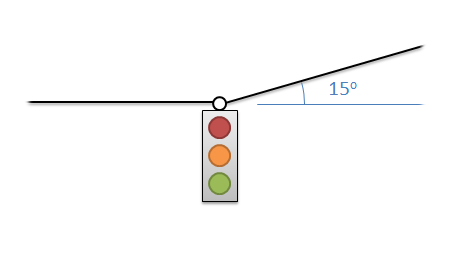### Question 2:

A 600 lb barrel is sitting in a groove as shown below. Determine normal forces acting on the barrel at points A and B.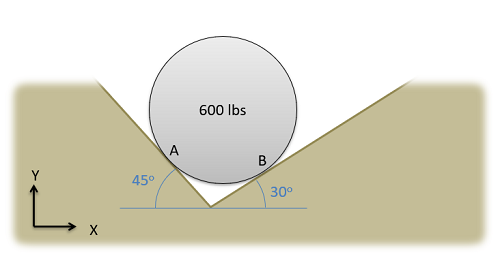### Question 3:

A cable supports a large mass resting along an angled surface. Determine the tension in the cable and the normal force acting on the mass.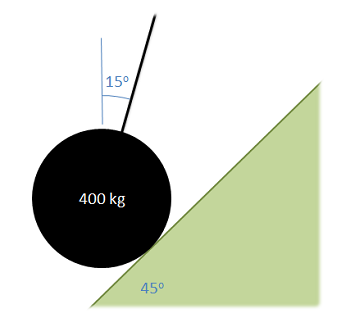### Question 4:

Three cables come together at the angles indicated. The tension in cable A is 50 lbs. Determine the tension in the other two cables.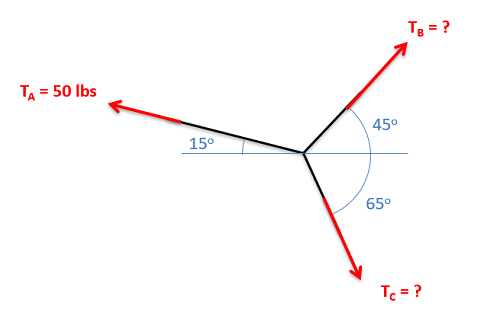### Question 5:

Two barrels are being carried on a handcart as shown below. Determine all forces acting on the bottom barrel.### Question 6:

Three soda cans, each weighing 0.75 lbs and having a diameter of 4 inches, are stacked in a formation as shown below. Assuming no friction forces, determine the normal forces acting on can B.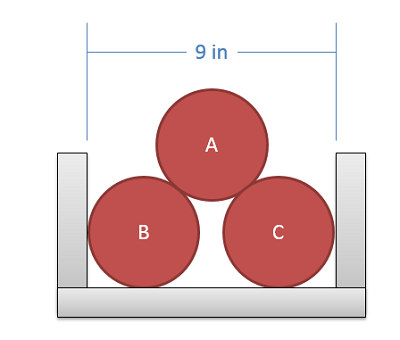### Question 7:

The SkyCam shown below is supported by three cables. Assuming it has a mass of 20kg and that it is currently in equilibrium find the tension in each of the three cables supporting the SkyCam.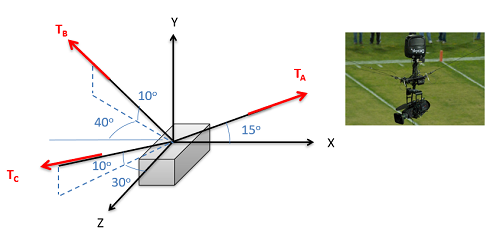Image by Jrienstra CC-BY-SA 3.0.

### Question 8:

A hot air balloon is tethered to the ground with three cables as shown below. If the balloon is pulling upwards with a net force of 900lbs, what is the tension in each of the three cables?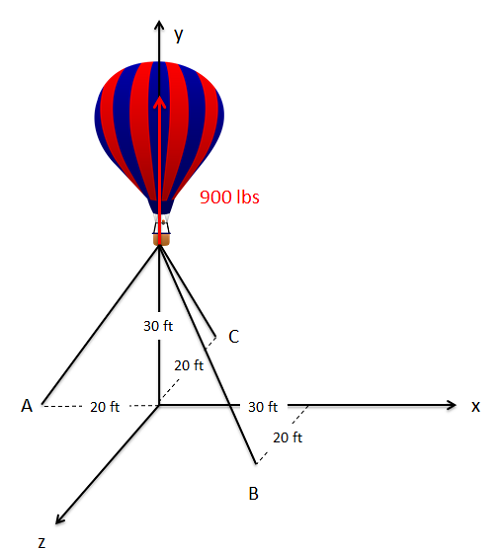Adapted from image by L. Aragon CC-BY-SA 3.0.